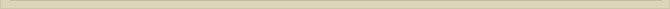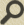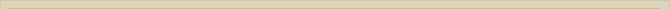## The Energy Level of the Hydrogen EM-free Rotator Atom

When you are interested in physics you must read “Unbelievable“!

Although it was so far not possible to obtain a mathematical solution for a EM-free rotator that resembles the hydrogen atom with it’s clear energy levels we continue the search.

When the electron circles around the proton at a smaller distance (R2<R1) the electrostatic energy (We) of the system is decreased according to: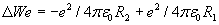(2)

The kinetic energy of the hydrogen atom, when considering the atom is an EM-rotator, would increase because the electron is now circling around with higher speed.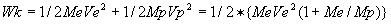(3)

Considering equation (1):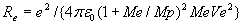we can express the dynamic/kinetic energy of the system Wk (3) with:(4)

Equation (2) shows the difference in electrostatic energy levels between the orbit radius R2 and R1. The electrostatic energy level of the atom, when R1= and R2=Re, is: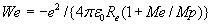(2a)

We observe that the kinetic energy of the system Wk (4) is at all times half of the released potential energy We of the electrostatic field (2a). The total energy of the system is the potential energy plus the kinetic energy: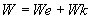(equation 2a+ equation 4)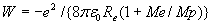(5)

When the atom emits a photon in our EM-rotator, due to the descent of the electron to a lower orbit, the energy of the photon is half the decreased potential energy. Because of the energy conservation law the energy of the emitted photon must be: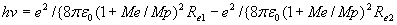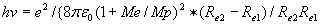These are the photons emitted the EM-rotator when the orbiting distances Re determines the energy level.

The energy level of the hydrogen atom, according to the Bohr-atomic model (Wb), is: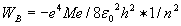(6) where n is the principal quantum number.

The Bohr-atomic model describes the observed energy levels of the atom very well for n=1,2,3… In equation (6) the only variable is n. The energy level of the EM-rotator (5), is completely determined the distance of the electron to the nucleus.

In classical EM-physics the energy level of the atom is completely determined the distance between nucleus and electron (5). The QM-solution (6) shows a quantified formula where the orbiting distance is no longer presented. Bohr’s Correspondence Principle tells us that both worlds (QM and EM) have to obey the same physic laws. However the quantum rules are of no significance in the macro-world.

At the quantum level physics have to obey the quantum-physics laws and the macrophysics laws. The Correspondence Principle of Bohr tells us that at the quantum level there are no additional rules, only that in the macro-world the quantum rules are no longer significant. The same principle tells us that the macrophysics laws also have to be valid at the quantum level.

Although the equations (5) en (6) are different Bohr’s Correspondence Principle tells us they could be the same; (5) describing the rules of the macro-world and (6) the rules of the micro-world where the laws of both worlds are relevant.

Neglecting the Me/Mp factor in equation (5) we get: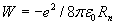(5a)  (**)

where Rn is the orbiting distance of the electron.

When equations (5a) and (6) are equally valid we can express the orbiting distance Re with the principal quantum number.

The energy of equation (6) must be equal to equation (5a) and therefore:(5c)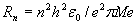(7)

For the ground level of the Bohr-hydrogen atom (n=1) the calculated distance, of course, coincides with the Bohr radius of the hydrogen atom Rb=5,29177.10^-11  meter; the orbiting distance of the electron being in ground state.

Similar calculations with the rydbergconstant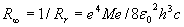and equation (5c) gives:(8)

The rydberg principal quantum number is calculated at.

The above calculations of the Bohr-radius of the hydrogen atom (n=1) and the rydberg quantum number are completely consistent with QM-calculations. Assuming that equation (5a) is identical to (6) doesn’t imply any discrepancy.

Summarizing we deducted that the EM-free rotator for the hydrogen atom has infinite solutions. With the assumption that the hydrogen atom is an EM-rotator there is at any distance or speed a possible equilibrium. The discrete energy levels of the electrons in the atom, the quantisation of energy, can completely be explained a quantum restriction that the electron can only have stable orbits around the nucleus at the discrete distances;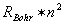.

Next chapter: The Quantisation of Distance